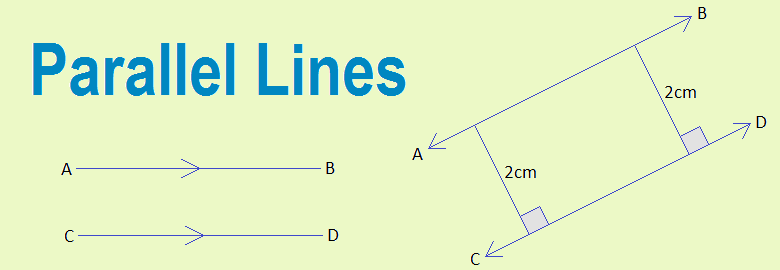# Parallel Lines## Parallel Lines

Two line segments are said to be parallel lines if they never meet to each other by producing from either side. Or, two line segments are said to be parallel lines if the perpendicular distance between them is same everywhere.
In the given figure AB and CD are parallel line segments as the perpendicular distance between AB and CD is 2cm everywhere and they never meet to each other by producing from either side.

Parallel lines are represented by the arrows on the line segments and written as AB//CD (read as AB parallel to CD) for two parallel line segments AB and CD given in the figure below.

### Construction of parallel lines using a set square

Step 1: Place a set square along the edge of a ruler and draw a line AB.
Step 2: Holding the ruler formly, slide the set square to the second position and draw a line segment MN.
Step 3: Then AB and MN are parallel
AB line segment is parallel with MN line segment.

### Construction of parallel lines using a compass

Step 1: Draw a line segment EF and take any point S above the line segment. Join E and S.
Step 2: Draw an equal arc at point E and S.
Step 3: Measure GH arc with a compass and cut an arc IJ from S so that the arc IT = the arc GH. Draw a line ST joining the points.
∴  TS is parallel to EF i.e TS//EF.

### Parallel Lines and Transversal

# When two parallel lines are cut by a transversal then the alternate angles are equal. In the given figure, AB and CD are parallel. So
i.      ∠AGH = ∠GHD
ii.    ∠BGH = ∠GHC

# When two parallel lines are cut by a transversal then corresponding angles are equal. In the given figure, MN and PQ are parallel. So,
i.      ∠RAM = ∠ABP
ii.    ∠MAB = ∠PBS
iii.   ∠RAN = ∠ABQ
iv.   ∠NAB = ∠QBS

# When two parallel lines are cut by a transversal then co-interior angles are supplementary. In the given figure, AB and CD are parallel. So,
i.      ∠AGH + ∠GHC = 180°
ii.    ∠BGH + ∠GHD = 180°

### Workout Examples

Example 1: Find the size of unknown angles.
Solution: From the figure,
x = 70° -----------------> Vertically opposite angles

x + y = 180° ------------> Co-interior angles
or,     70° + y = 180°
or,     y = 180° - 70°
or,     y = 110°

z = x ---------------> Alternate angles
= 70°

x = 70°, y = 110° and z = 70°

Example 2: Find the size of unknown angles.
Solution: Here,
A line PQ constructed through the point F which is parallel to AB or CD.
EFQ + 120° = 180° ---------------> Co-interior angles
or,     EFQ = 180° - 120°
or,     EFQ = 60°

GFQ = 30° -------------------> Alternate angles

x = EFQ + GFQ
= 60° + 30°
= 90°

Example 3: Find the size of unknown angles.
Solution: From the figure,
a = 66° -----------------> Alternate angles

a + b = 180° -------------------> Co-interior angles
or,     66° + b = 180°
or,     b = 180° - 66°
or,     b = 114°

c = b -----------------------> Alternate angles
= 114°

a = 66°, b = 114° and c = 114°

You can comment your questions or problems regarding the parallel lines here.